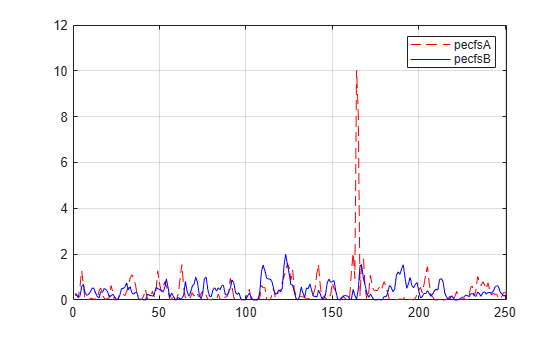# wdecenergy

Multisignal 1-D decomposition energy distribution

## Syntax

```[E,PEC,PECFS] = wdecenergy(DEC) [E,PEC,PECFS,IDXSORT,LONGS] = wdecenergy(DEC,'sort') [E,PEC,PECFS] = wdecenergy(DEC,OPTSORT,IDXSIG) [E,PEC,PECFS,IDXSORT,LONGS] = wdecenergy(DEC,OPTSORT,IDXSIG) ```

## Description

`[E,PEC,PECFS] = wdecenergy(DEC)` computes the vector E that contains the energy (L2-Norm) of each decomposed signal, the matrix PEC that contains the percentage of energy for each wavelet component (approximation and details) of each signal, and the matrix PECFS that contains the percentage of energy for each coefficient.

• E(i) is the energy (L2-norm) of the ith signal.

• PEC(i,1) is the percentage of energy for the approximation of level MAXLEV = DEC.level of the ith signal.

• PEC(i,j), j=2,...,MAXLEV+1 is the percentage of energy for the detail of level (MAXLEV+1-j) of the ith signal.

• PECFS(i,j), is the percentage of energy for jth coefficients of the ith signal.

`[E,PEC,PECFS,IDXSORT,LONGS] = wdecenergy(DEC,'sort')` returns PECFS sorted (by row) in ascending order and an index vector IDXSORT.

• Replacing 'sort' by 'ascend' returns the same result.

• Replacing 'sort' by 'descend' returns PECFS sorted in descending order.

LONGS is a vector containing the lengths of each family of coefficients.

`[E,PEC,PECFS] = wdecenergy(DEC,OPTSORT,IDXSIG)` returns the values for the signals whose indices are given by the `IDXSIG` vector.

`[E,PEC,PECFS,IDXSORT,LONGS] = wdecenergy(DEC,OPTSORT,IDXSIG)` returns the values for the signals whose indices are given by the IDXSIG vector, the index vector IDXSORT, and LONGS, which is a vector containing the lengths of each family of coefficients. Valid values for OPTSORT are 'none', 'sort', 'ascend', 'descend'.

## Examples

collapse all

Load the 23 channel EEG data `Espiga3` . The channels are arranged column-wise. The data is sampled at 200 Hz.

`load Espiga3`

Perform a decomposition at level 2 using the `db2` wavelet.

`dec = mdwtdec('c',Espiga3,2,'db2')`
```dec = struct with fields: dirDec: 'c' level: 2 wname: 'db2' dwtFilters: [1x1 struct] dwtEXTM: 'sym' dwtShift: 0 dataSize: [995 23] ca: [251x23 double] cd: {[499x23 double] [251x23 double]} ```

Compute the energy distribution.

`[e,pec,pecfs] = wdecenergy(dec);`

Display the total energy and the distribution of energy for each wavelet component (A2, D2, D1) in the second channel.

```idx = 2; e(idx)```
```ans = 8.0761e+05 ```
`perA2D2D1 = pec(idx,:)`
```perA2D2D1 = 1×3 99.0583 0.8535 0.0882 ```

Compare the coefficient energy distribution for signal 1 and signal 10. Because most of the energy is in the approximation coefficients, zoom in the x-axis by the number of approximation coefficients.

```sigA = 1; sigB = 10; pecfsA = pecfs(sigA,:); pecfsB = pecfs(sigB,:); plot(pecfsA,'r--') hold on plot(pecfsB,'b') grid on legend('pecfsA','pecfsB') xlim([0 size(dec.ca,1)])```Mesa, Hector. “Adapted Wavelets for Pattern Detection.” In Progress in Pattern Recognition, Image Analysis and Applications, edited by Alberto Sanfeliu and Manuel Lazo Cortés, 3773:933–44. Berlin, Heidelberg: Springer Berlin Heidelberg, 2005. https://doi.org/10.1007/11578079_96.